Related Articles

# Tricks To Solve Mixture and Alligation

Mixture and alligation are one of the most important and intrinsic topics for SSC CGL. As it is not an individual chapter, it is a method of solving problems of different topics like ratio, profit, loss, average, etc. Almost 3-4 questions are asked in the prelims exam that can be solved using the technique of allegation. In this article we will be studying the method of the allegation and how can we implement it for solving questions of various topics in a much easier and smarter way.

Mixture: It is the process of mixing two or more elements/ingredients together.

Allegation: It is a process or a rule that tells us how to mix two or more ingredients/mixtures to get the desired mixture of a certain price & concentration.

Now there are 2 ways of applying the allegation technique to questions.

## Formula Method:

Quantity of cheaper/ Quantity of dearer = (Price of dearer – Mean price)/ (Mean Price- Price of cheaper)

## Diagram Method:

The above-mentioned formula can be better understood using the diagram method & it is more convenient to use this method.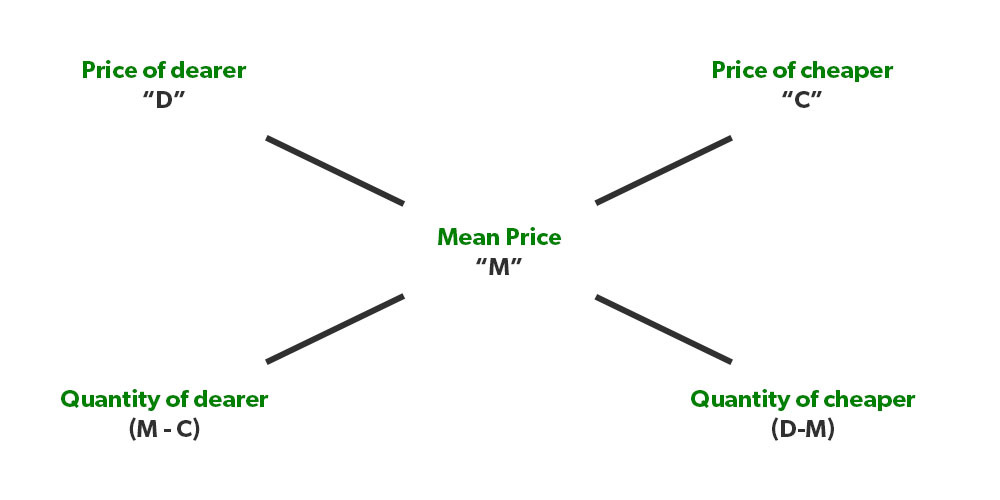#### Note 2.  (D-M)/(M-C) is nothing but the ratio of quantities of both the items, so even if we are given a ratio in which 2 items are mixed, we can find the “M”.

Now let’s try to solve a variety of problems using the allegation method which will help you in understanding the concept in a much better way.

Illustration 1. If 20 kgs of rice which cost 45 Rs/Kg is mixed with 25 kgs of rice which costs 30 Rs/Kg. What will be the price of the final mixture?

Solution: In this question, we have to find “M”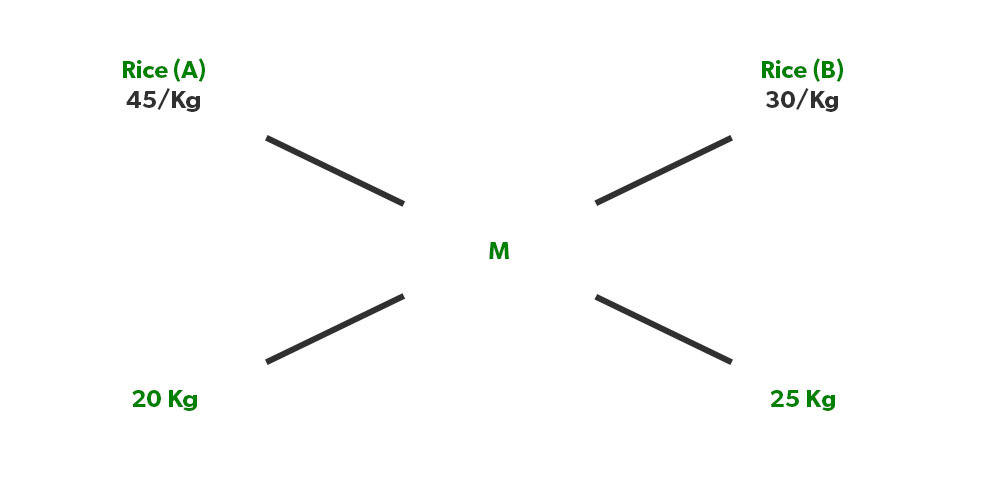Now as we know, (45-M)/(M-30)=25/20

⇒ (45-M)/(M-30)= 5/4

⇒180-4M = 5M- 150

⇒ 330= 9M

⇒ M= 330/9= 36.6 Rs/Kg

Illustration 2. Oranges of type A that cost 60 Rs/Kg is mixed with the orange of type B that costs 80 Rs/Kg in a ratio of 3:5. What will be the price of the resultant mixture of oranges?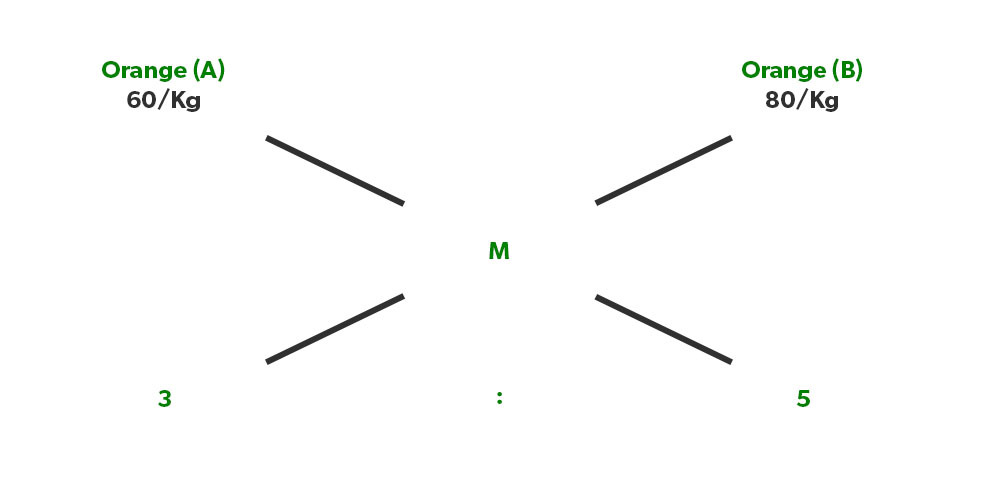Solution: Again applying the same formula,

(60-M)/(M-80)= 5/3

180-3M = 5M-400

580= 8M

M= 580/8= 72.5 Rs

Illustration 3. If rice or 45 Rs/kg is mixed with rice of 35 Rs/kg and the price of the resultant mixture is 42.5 Rs/kg. What is the ratio that they were mixed in?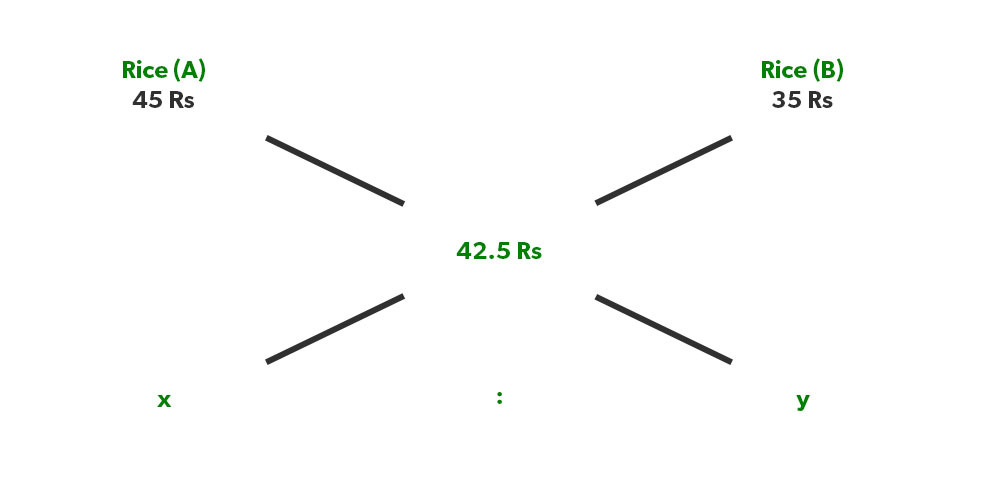Solution: As we know, (45-42.5)/(42.5-35) = Y/X

2.5/7.5= Y/X

1/3 = Y/X, hence they were mixed in the ratio of 3:1.

Illustration 4. Mixture A which contains 30% water is mixed with Mixture B which has 20% water in a ratio of 2:5. What will be the % of water in the resultant mixture?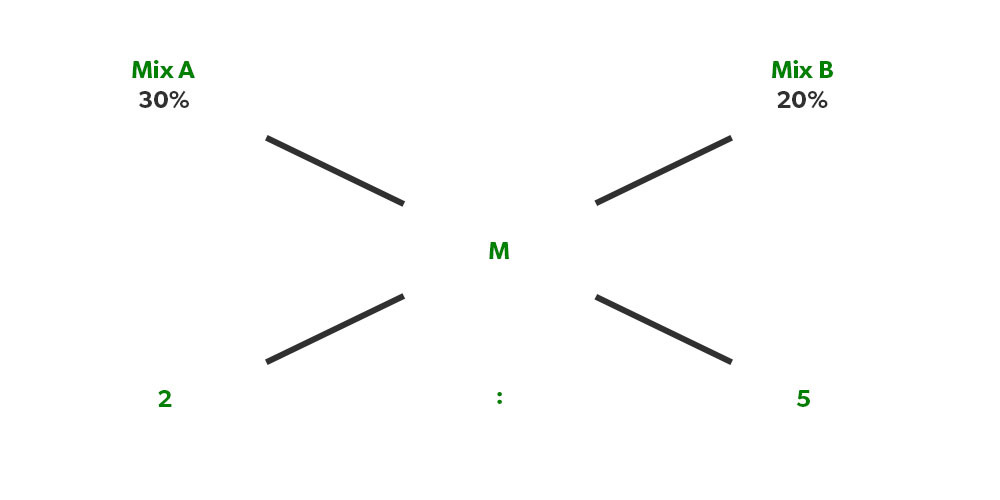Solution: Allegation can also be applied to percentages & not just absolute values.

(30%-M)/(M-20%)= 5/2

60% – 2M= 5M – 100%

160% = 7M

M = 160%/7 = 22.8%

Illustration 5. A shopkeeper sells one item at 20% profit and another item at 5% profit. If the overall profit of the shopkeeper is 10 per cent. What is the ratio of the CP of item A to item B?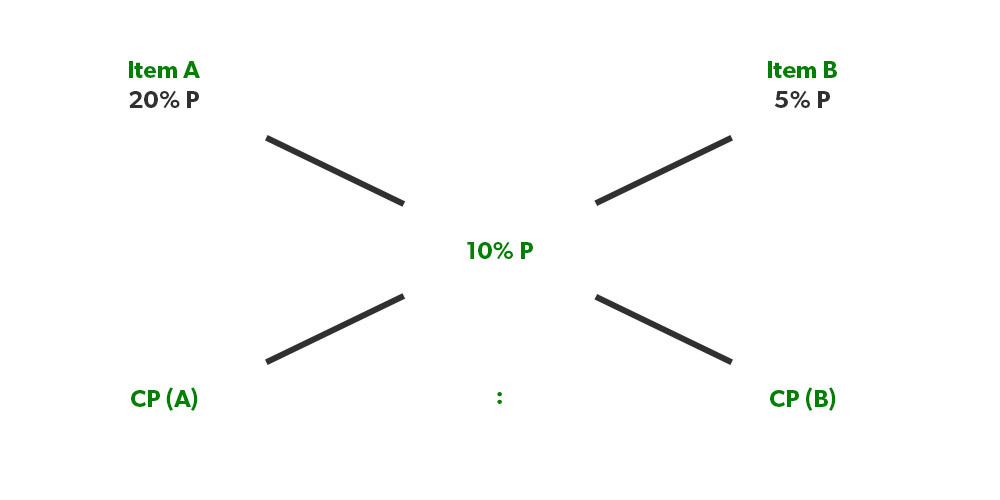Solution: Here, the ratio of quantities will give us ratio of the cost price

So, (20%-10%)/ (10%-5%)= CP (B)/CP (A)

10%/5%= CP (B)/ CP (A)

2:1 = CP (A)/ CP(B)

Illustration 6. A shopkeeper sells Type A rice at 40 Rs/Kg and types B rice at 60 Rs/kg. If he wants to gain a profit of 10% after selling the mixture of both types in the ratio Of 2:3. What should be the selling price of the mixture?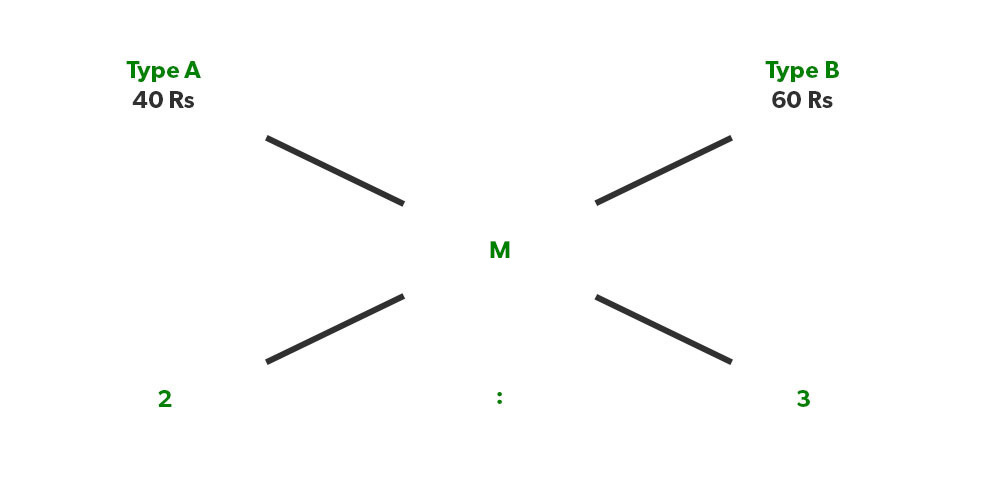Solution: Here the value of M will give us no profit no loss amount.
(40-M)/(M-60)= 3/2

It gives us M= 260/5 = 52

Now to get a profit of 10 %, the shopkeeper has to sell it at, (52) × (110/100) = 57.2 Rs

Illustration 7. If a shopkeeper sells an item of 2000 Rs at 15 per cent profit & another item of 3500 Rs at 5 per cent loss. Find his overall profit/loss percentage?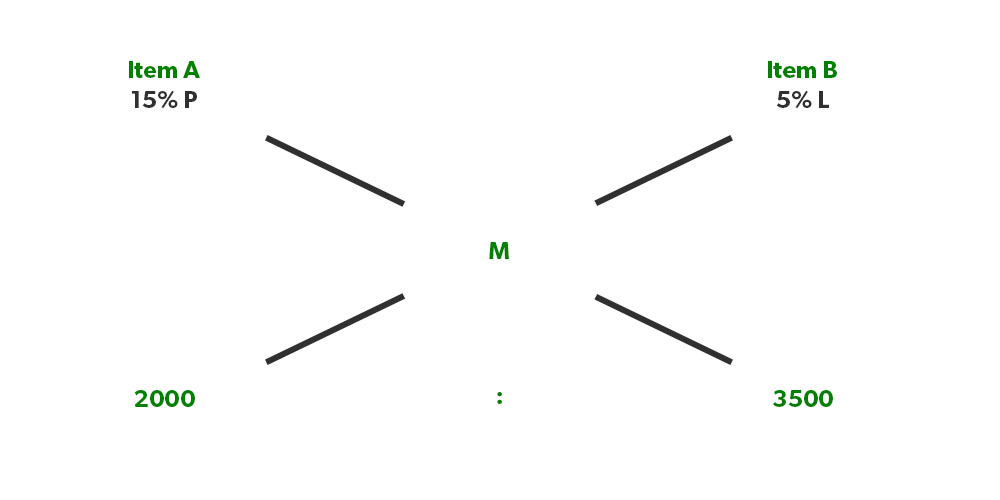Solution: We will take loss % with minus sign,

(15% – M)/ (M- (-5%) = 7/4

It gives us M = 2.27%

Illustration 8. If the average marks of boys in a class is 75 and the average marks of girls in that class is 80. If the ratio of boys to girls in that class is 4:3. Find the average marks of the class?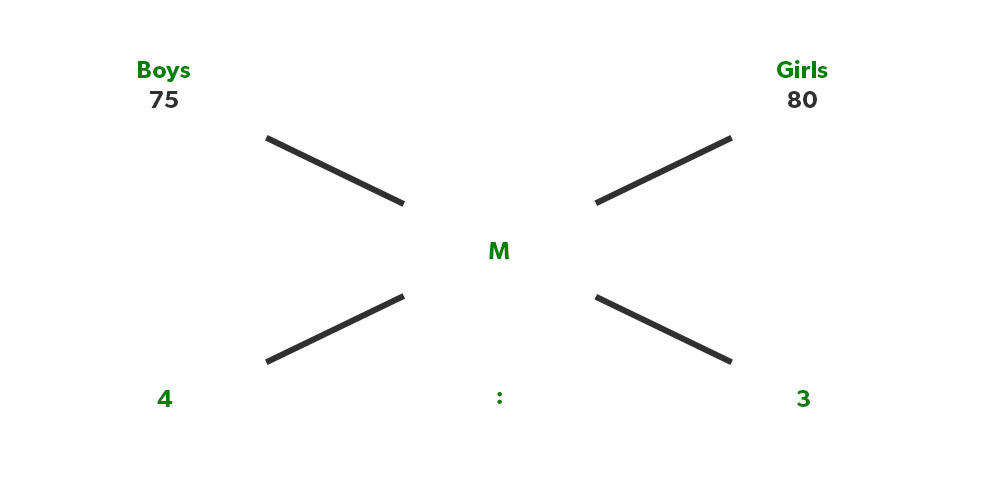Solution: Here also, we can find the average of the whole class easily,

(75-M)/( M-80)= 3/4

M = 77.14

Illustration 9. If a shopkeeper sold a mixture of mangoes at 100 Rs/Kg in which he mixed 2 types of mangoes that cost him 50 Rs/Kg and 75 Rs/Kg in the ratios of 2:3 respectively. What is the profit percentage of the shopkeeper?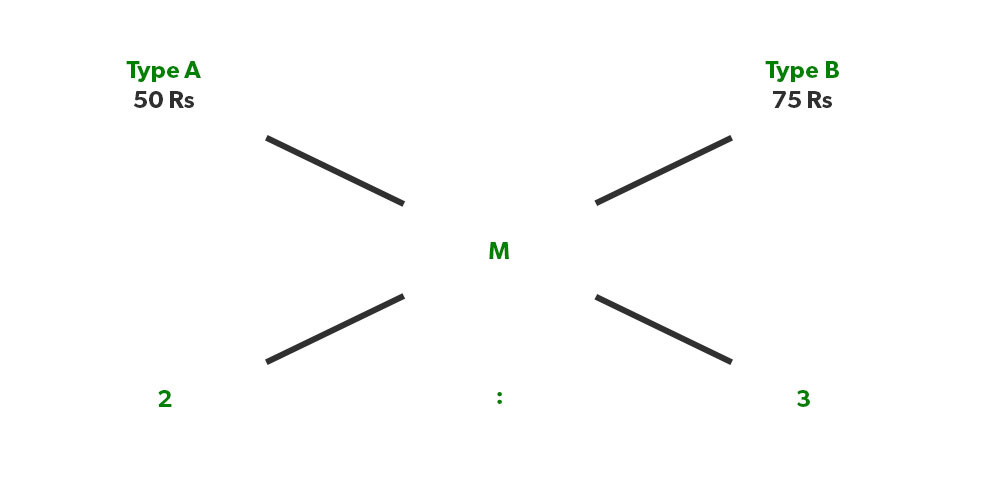Solution: (50-M)/( M-75)= 3/2

M= 65 Rs/kg

Now he sold this at 100 Rs/Kg, so his profit is (35/65)× 100 = 53.8 % P

Illustration 10.  If a cyclist covered 3/5th distance or the track with 15 km/hr and the rest of the distance with 10km/her. What is the average speed of the cyclist?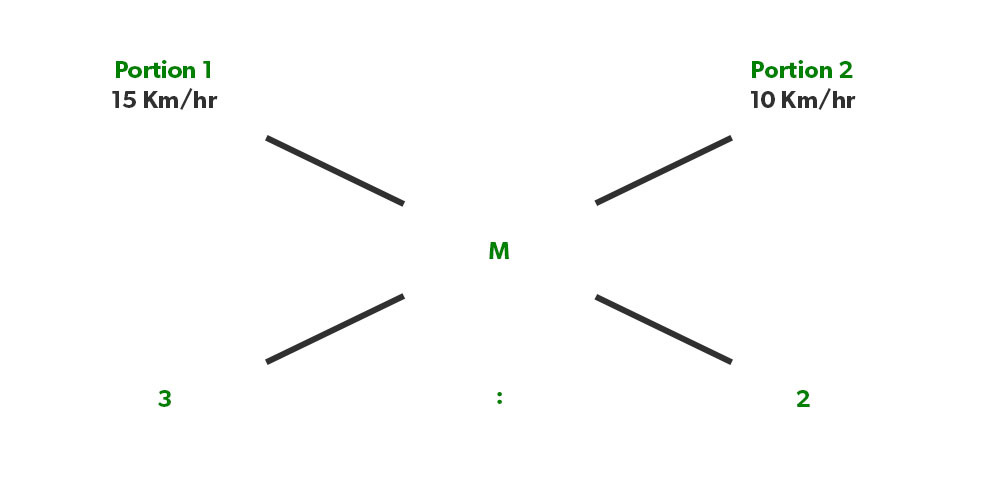Solution: (15-M)/(M-10)= 2/3

M= 13 km/Hr

Illustration 11. In a village, the average age of males is 35 years & in another village, the average age of males is 30 years. If the ratio of the total number of females in both the villages is 2:3. Find the average age if both the villages are combined?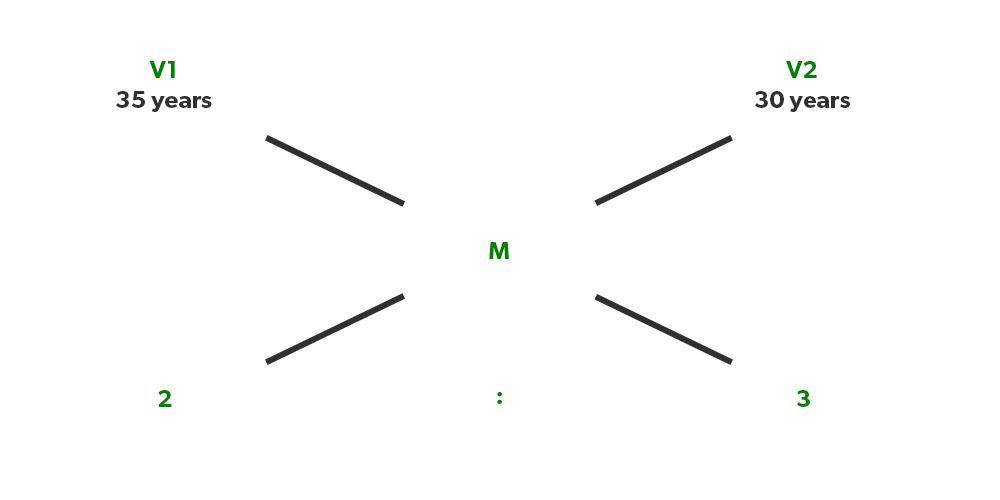Solution: Average age will be, (35-M)/ (M-30)= 3/2

That gives us M= 36 Years

Illustration 12. A lender gives money at 8% SI for 2 years to a guy & takes money from somewhere at 5% SI for 3 years. If the amount he lent to the amount he took is in the ratio of 3:4. Find his overall profit/loss %?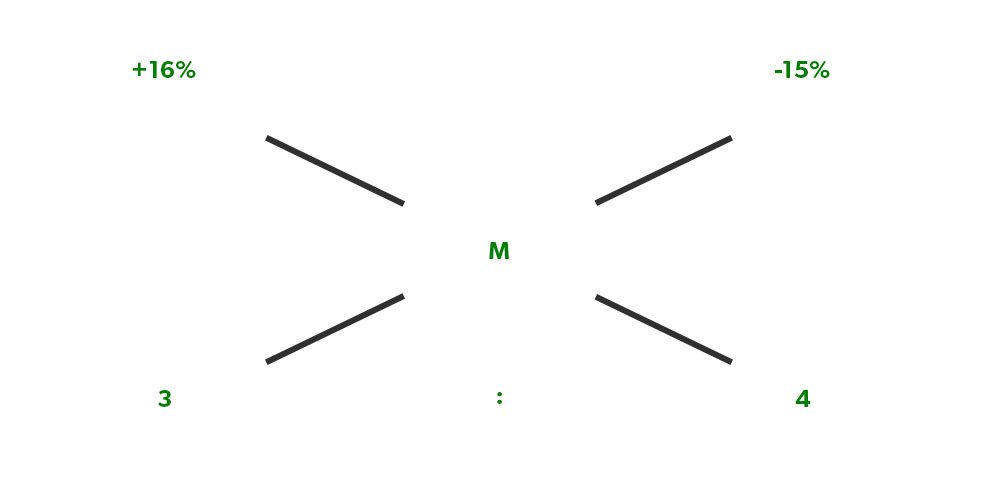Solution: 8% for 2 years SI becomes 16% & 5% for 3 years SI becomes 15%.

overall profit/loss % will be, (16%- M)/ (M+15%)= 4/3

That gives us M= -12/7% = loss of 12/7%

Illustration 13. If a racer can finish a track in 10 hours & another racer can finish the same track in 15 hours. If both are supposed to take part in the relay race & racer 1 has to run the 2/5th part of the track. How long will it take for both of them to complete the race?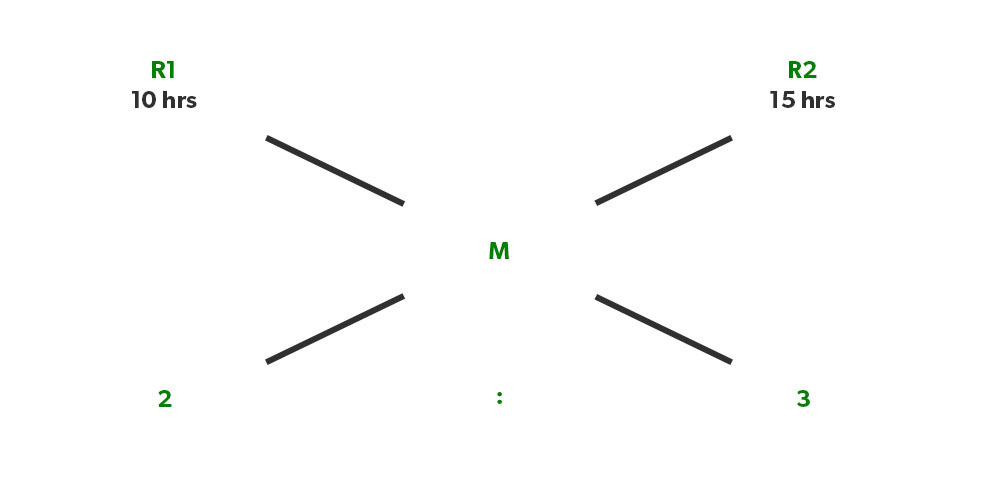Solution: If racer 1 is covering 2/5th of the track, hence racer 2 will cover 3/5th of the track.

Applying the rule of the allegation, we get

(10 Hrs – M)/ (M-15 Hrs)= 3/2

This gives us, M= 13 Hrs

Illustration 14. A shopkeeper mixes water in milk If he mixes 1-litre water in 5-litre milk & the actual cost of milk is 30Rs/Litre but he sells the milk at 28 Rs/litre. Find his actual profit or loss?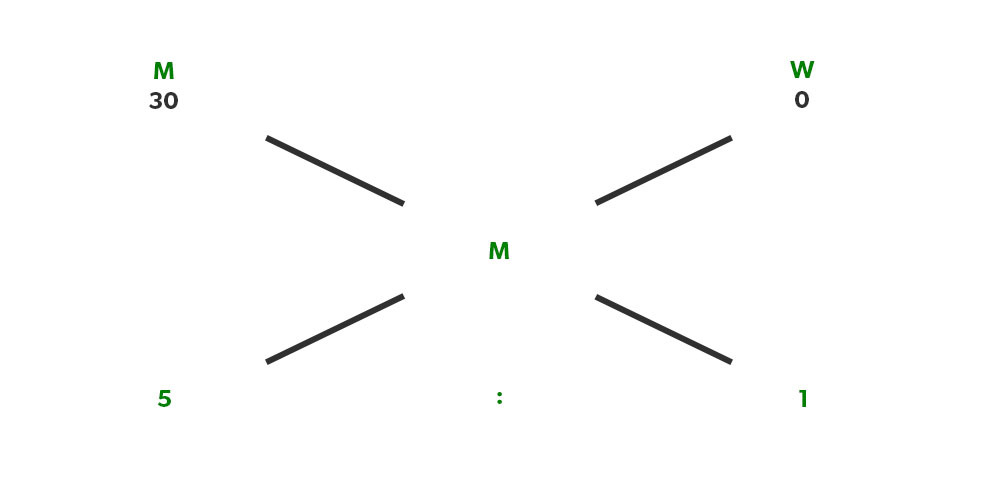Solution: The key concept here to understand is that water costs zero rupees.

Hence the no profit no loss value of the mixture is,

(30-M)/ (M-0)= 1/5

That gives us M= 25 Rs.

Now he sells this at 28 Rs, so profit % is (3/25) × 100 = 12%

Illustration 15. A guy who works at a juice centre mixes water with juice in a ratio of 5:1 respectively. If the actual price of fruits is 50 Rs/glass & he sells 60 Rs/glass. find his actual profit/glass?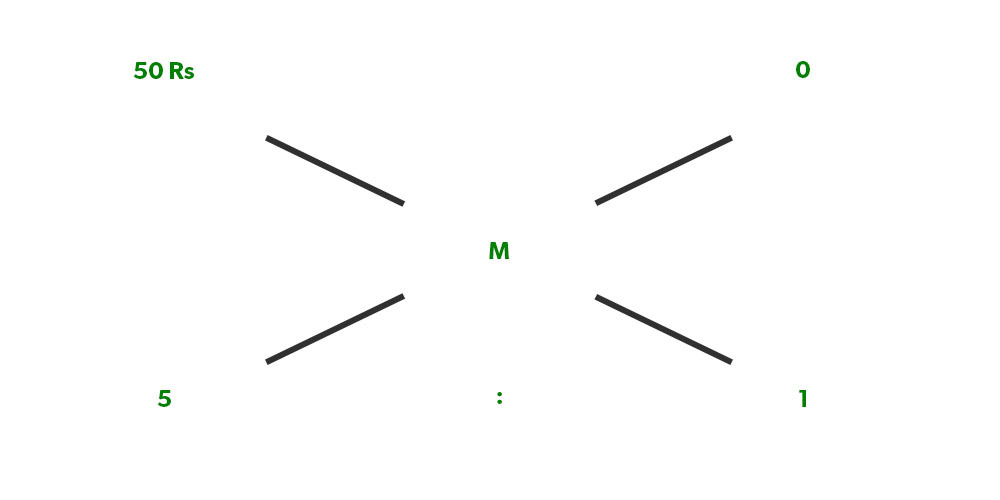Solution: Again, repeating the same logic

(50-M)/ (M-0)= 1/5

M= 250/6 Rs, so his profit % is [60- (250/6)]/ (250/6) × 100 = 44%

This was some illustration to help you understand the application of Mixture & Allegation.

Whether you're preparing for your first job interview or aiming to upskill in this ever-evolving tech landscape, GeeksforGeeks Courses are your key to success. We provide top-quality content at affordable prices, all geared towards accelerating your growth in a time-bound manner. Join the millions we've already empowered, and we're here to do the same for you. Don't miss out - check it out now!

Previous
Next